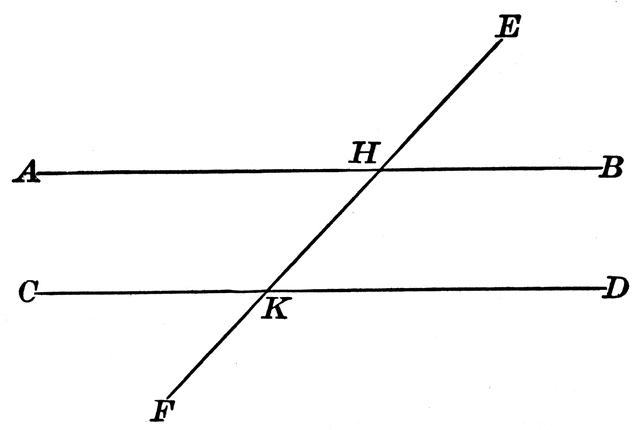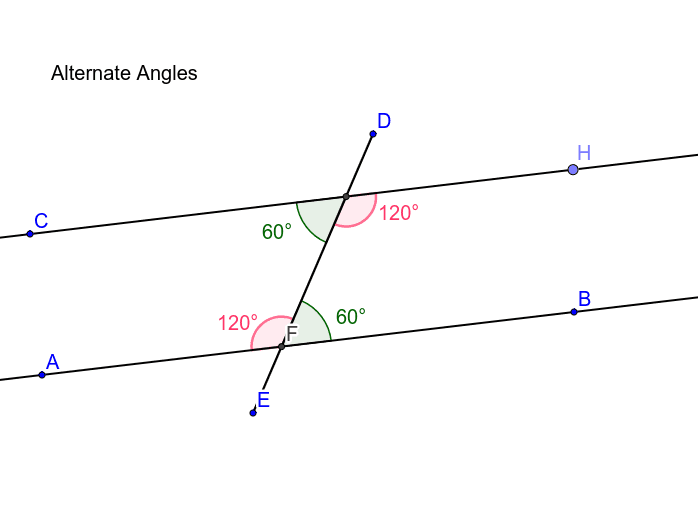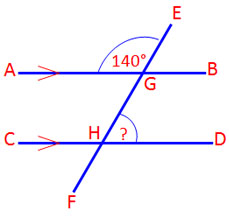# Parallel  lines

## What  is  parallel  lines ?In geometryparallel lines are lines in a plane which do not meet; that is, two lines in a plane that do not intersect or touch each other at any point are said to be parallel. By extension, a line and a plane, or two planes, in three-dimensional Euclidean space that do not share a point are said to be parallel. However, two lines in three-dimensional space which do not meet must be in a common plane to be considered parallel; otherwise they are called skew lines. Parallel planes are planes in the same three-dimensional space that never meet.

Parallel lines are the subject of Euclid‘s parallel postulate. Parallelism is primarily a property of affine geometries and Euclidean geometry is a special instance of this type of geometry. In some other geometries, such as hyperbolic geometry, lines can have analogous properties that are referred to as parallelism.

## What  is  alterate  angles?Alternate angles are one of the special kinds of angles in geometry. These are the set of non adjacent angles for either sides of the transversal. Alternate angles are shaped by two parallel lines crossed by the transversal.

## What  is  corners  theorem ?In mathematics, the corners theorem is a result, proved by Miklós Ajtai and Endre Szemerédi, of a statement in arithmetic combinatorics. It states that for every ε > 0 there exists N such that given at least εN2 points in the N × N grid {1, …, N} × {1, …, N}, there exists a corner, i.e., three points in the form (xy), (x + hy), and (xy + h). Later, Solymosi (2003) gave a simpler proof, based on the triangle removal lemma. The corners theorem implies Roth’s theorem.

## 4 thoughts on “Parallel lines”

1. Pingback: Online Shopping

2. Pingback: 바카라사이트

3. Pingback: tile ca cuoc macao

4. Pingback: keo bon da